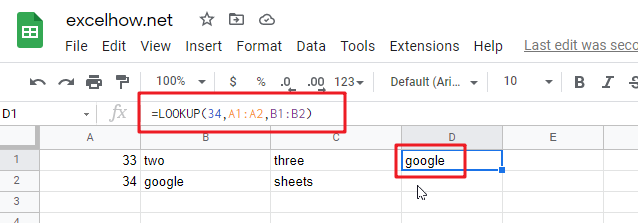# ExcelHow

This post will guide you how to use Google Sheets LOOKUP function with syntax and examples.

## Description

The Google Sheets LOOKUP function looks up a value through a sorted row or column and returns the corresponding value from another row or column.

The LOOKUP function can be used to lookup a value in a one-row or one-column range in google sheets.

The LOOKUP function is a build-in function in Google Sheets and it is categorized as a Lookup function.

## Syntax

The syntax of the LOOKUP function is as below:

= LOOKUP (lookup_value, lookup_vector, [result_vector])

Where the LOOKUP function arguments are:

• Lookup_value -This is a required argument. A value that you want to search in the lookup_vector.
• Lookup_vector – This is a required argument. A Range that only contains one row or one column.
• Result_vector – This is an optional argument. A Range that only contains one row or one column. It must be the same size as Lookup_vector. The lookup function will look up the value in the lookup_value range and returns the value from the same position in the result_vector.

Note:

• If `result_vector` is omitted, the Lookup function will return the first column data.
• LOOKUP function will only work If data in lookup_vector is sorted. And this function assumes that `lookup_vector` is sorted in ascending order.
• When `lookup_value` is not found, LOOKUP function will return the next smallest value in the same row or column.
• When `lookup_value` is greater than all values in `lookup_vector`, LOOKUP function will return the last value.
• When `lookup_value` is less than the first value in `lookup_vector`, LOOKUP function will return the `#N/A` error.
• Result_vector must be only a single row or column.

## Google Sheets LOOKUP Function Examples

The below examples will show you how to use google sheets LOOKUP Function to search a value from a vector or array.

#1 To look up the value “34” in A1:A2 range, then get the value from the same row or column in B1:B2 range, just using the following google sheets formula:

`=LOOKUP(34,A1:A2,B1:B2)`